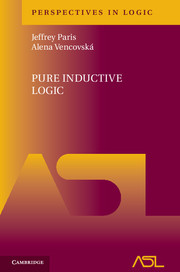# Pure Inductive Logic

## Jeffrey Paris and Alena VencovskaYear: 2015
ISBN-13: 9781107042308
354 pages. Hardcover.
Buy now (when ordering, include the discount code ASL2016 to receive the 25% ASL member discount)

Pure inductive logic is the study of rational probability treated as a branch of mathematical logic. This monograph, the first devoted to this approach, brings together the key results from the past seventy years plus the main contributions of the authors and their collaborators over the last decade to present a comprehensive account of the discipline within a single unified context. The exposition is structured around the traditional bases of rationality, such as avoiding Dutch Books, respecting symmetry and ignoring irrelevant information. The authors uncover further rationality concepts, both in the unary and in the newly emerging polyadic languages, such as conformity, spectrum exchangeability, similarity and language invariance. For logicians with a mathematical grounding, this book provides a complete self-contained course on the subject, taking the reader from the basics up to the most recent developments. It is also a useful reference for a wider audience from philosophy and computer science.

• The first book to comprehensively treat inductive logic as a branch of mathematical logic, with many new existing results collected together into one unified presentation
• A self-contained introduction to the field that takes the reader from the basics right through to the forefront of current research
• Can also be used as an accessible work of reference for philosophers and computer scientists, as well as mathematical logic

Part I. The Basics:

1. Introduction to pure inductive logic
2. Context
3. Probability functions
4. Conditional probability
5. The Dutch book argument
6. Some basic principles
7. Specifying probability functions

Part II. Unary Inductive Logic:

1. Introduction to unary pure inductive logic
2. de Finetti’s representation theorem
3. Regularity and universal certainty
4. Relevance
5. Asymptotic conditional probabilities
6. The conditionalization theorem
7. Atom exchangeability
8. Carnap’s continuum of inductive methods
9. Irrelevance
10. Another continuum of inductive methods
11. The NP-continuum
12. The weak irrelevance principle
13. Equalities and inequalities
14. Principles of analogy
15. Unary symmetry

1. Introduction to polyadic pure inductive logic
4. Spectrum exchangeability
5. Conformity
6. The probability functions $u^{\overline{p},L}$
7. The homogeneous/heterogeneous divide
8. Representation theorems for Sx
9. Language invariance with Sx
10. Sx without language invariance
11. A general representation theorem for Sx
12. The Carnap–Stegmüller principle
13. Instantial relevance and Sx
14. Equality
15. The polyadic Johnson’s sufficientness postulate
19. The functions $u_{\overline{E}}^{\overline{p},L}$